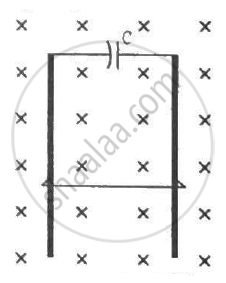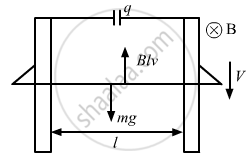Advertisement Remove all ads

# A Wire of Mass M And Length L Can Slide Freely on a Pair of Smooth, Vertical Rails (Figure). a Magnetic Field B Exists in the Region in the Direction Perpendicular to the Plane of the Rails. - Physics

Sum

A wire of mass m and length l can slide freely on a pair of smooth, vertical rails (figure). A magnetic field B exists in the region in the direction perpendicular to the plane of the rails. The rails are connected at the top end by a capacitor of capacitance C. Find the acceleration of the wire neglecting any electric resistance.Advertisement Remove all ads

#### Solution

Let the velocity of the rod at an instant be v and the charge on the capacitor be q.The emf induced in the rod is given by

e = Blv

The potential difference across the terminals of the capacitor and the ends of the rod must be the same, as they are in parallel.

$\therefore\frac{q}{C} = Blv$

And,

q = C × Blv = CBlv

Current in the circuit:-

$i = \frac{dq}{dt} = \frac{d(CBlv)}{dt}$

$\Rightarrow i = CBl\frac{dv}{dt} = CBla$        ........(a = acceleration of the rod)

The force on the rod due to the magnetic field and its weight are in opposite directions.

∴ mg − ilB = ma

⇒ mg − cBla × lB = ma

⇒ ma + cB2l2a = mg

⇒ a(m + cB2l2) = mg

$\Rightarrow a = \frac{mg}{m + c B^2 l^2}$

Concept: Induced Emf and Current
Is there an error in this question or solution?
Advertisement Remove all ads

#### APPEARS IN

HC Verma Class 11, Class 12 Concepts of Physics Vol. 2
Chapter 16 Electromagnetic Induction
Q 63 | Page 311
Advertisement Remove all ads

#### Video TutorialsVIEW ALL 

Advertisement Remove all ads
Share
Notifications

View all notifications

Forgot password?